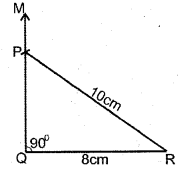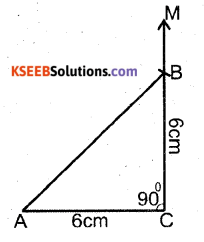# KSEEB Solutions for Class 7 Maths Chapter 10 Practical Geometry Ex 10.5

Students can Download Chapter 10 Practical Geometry Ex 10.5, Question and Answers, Notes Pdf, KSEEB Solutions for Class 7 Maths, Karnataka State Board Solutions help you to revise complete Syllabus and score more marks in your examinations.

## Karnataka State Syllabus Class 7 Maths Chapter 10 Practical Geometry Ex 10.5

Question 1.
Construct the right angled ∆ PQR? where m∠Q = 90°, QR = 8 cm and PR = 10 cm.
Solution:Solution:
Steps of Construction

1. Draw a line segment of length 8 cm and named as QR. At Q draw QM ⊥ QR.
2. With R as centre, draw an arc of radius 10 cm and cut the ⊥le line at P.
3. Join PR. Now we get the required PQR ∆Question 2.
Construct a right-angled triangle whose hypotenuse is 6 cm long and one of the legs is 4
cm long.
Solution:Steps of Construction

1. Draw a line segment of length 4 cm and named it AB. At A draw a ⊥ line AM.
2. With B as centre, draw an arc of 6 cms cut the x line at ‘C ’. Now we obtained the required triangle ABC.Question 3.
Construct an isosceles right-angled triangle ABC, where m∠ACB = 90° and AC = 6 cm.
Solution:Steps of Construction

1. Draw a line segment of 6 cm, ie., AC. At C draw CM ⊥ CA.
2. With ‘C’ as the centre draw an arc of radius 6 cm to intersect CM at ‘B’
3. Join AB. Now we get the required ∆ACB.
error: Content is protected !!